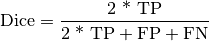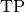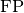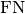Shortcuts

# Dice¶

## Module Interface¶

class torchmetrics.Dice(zero_division=0, num_classes=None, threshold=0.5, average='micro', mdmc_average='global', ignore_index=None, top_k=None, multiclass=None, **kwargs)[source]

Computes Dice:Whereandrepresent the number of true positives and false positives respecitively.

It is recommend set ignore_index to index of background class.

The reduction method (how the precision scores are aggregated) is controlled by the average parameter, and additionally by the mdmc_average parameter in the multi-dimensional multi-class case.

As input to forward and update the metric accepts the following input:

As output to forward and compute the metric returns the following output:

• dice (Tensor): A tensor containing the dice score.

• If average in ['micro', 'macro', 'weighted', 'samples'], a one-element tensor will be returned

• If average in ['none', None], the shape will be (C,), where C stands for the number of classes

Parameters
• num_classes – Number of classes. Necessary for 'macro', 'weighted' and None average methods.

• threshold – Threshold for transforming probability or logit predictions to binary (0,1) predictions, in the case of binary or multi-label inputs. Default value of 0.5 corresponds to input being probabilities.

• zero_division – The value to use for the score if denominator equals zero.

• average

Defines the reduction that is applied. Should be one of the following:

• 'micro' [default]: Calculate the metric globally, across all samples and classes.

• 'macro': Calculate the metric for each class separately, and average the metrics across classes (with equal weights for each class).

• 'weighted': Calculate the metric for each class separately, and average the metrics across classes, weighting each class by its support (tp + fn).

• 'none' or None: Calculate the metric for each class separately, and return the metric for every class.

• 'samples': Calculate the metric for each sample, and average the metrics across samples (with equal weights for each sample).

Note

What is considered a sample in the multi-dimensional multi-class case depends on the value of mdmc_average.

• mdmc_average

Defines how averaging is done for multi-dimensional multi-class inputs (on top of the average parameter). Should be one of the following:

• None [default]: Should be left unchanged if your data is not multi-dimensional multi-class.

• 'samplewise': In this case, the statistics are computed separately for each sample on the N axis, and then averaged over samples. The computation for each sample is done by treating the flattened extra axes ... as the N dimension within the sample, and computing the metric for the sample based on that.

• 'global': In this case the N and ... dimensions of the inputs are flattened into a new N_X sample axis, i.e. the inputs are treated as if they were (N_X, C). From here on the average parameter applies as usual.

• ignore_index – Integer specifying a target class to ignore. If given, this class index does not contribute to the returned score, regardless of reduction method. If an index is ignored, and average=None or 'none', the score for the ignored class will be returned as nan.

• top_k – Number of the highest probability or logit score predictions considered finding the correct label, relevant only for (multi-dimensional) multi-class inputs. The default value (None) will be interpreted as 1 for these inputs. Should be left at default (None) for all other types of inputs.

• multiclass – Used only in certain special cases, where you want to treat inputs as a different type than what they appear to be.

Raises
• ValueError – If average is none of "micro", "macro", "weighted", "samples", "none", None.

• ValueError – If mdmc_average is not one of None, "samplewise", "global".

• ValueError – If average is set but num_classes is not provided.

• ValueError – If num_classes is set and ignore_index is not in the range [0, num_classes).

Example

>>> import torch
>>> from torchmetrics import Dice
>>> preds  = torch.tensor([2, 0, 2, 1])
>>> target = torch.tensor([1, 1, 2, 0])
>>> dice = Dice(average='micro')
>>> dice(preds, target)
tensor(0.2500)


Initializes internal Module state, shared by both nn.Module and ScriptModule.

## Functional Interface¶

torchmetrics.functional.dice(preds, target, zero_division=0, average='micro', mdmc_average='global', threshold=0.5, top_k=None, num_classes=None, multiclass=None, ignore_index=None)[source]

Computes Dice:Whereandrepresent the number of true positives and false negatives respecitively.

It is recommend set ignore_index to index of background class.

The reduction method (how the recall scores are aggregated) is controlled by the average parameter, and additionally by the mdmc_average parameter in the multi-dimensional multi-class case.

Parameters
• preds (Tensor) – Predictions from model (probabilities, logits or labels)

• target (Tensor) – Ground truth values

• zero_division (int) – The value to use for the score if denominator equals zero

• average (Optional[str]) –

Defines the reduction that is applied. Should be one of the following:

• 'micro' [default]: Calculate the metric globally, across all samples and classes.

• 'macro': Calculate the metric for each class separately, and average the metrics across classes (with equal weights for each class).

• 'weighted': Calculate the metric for each class separately, and average the metrics across classes, weighting each class by its support (tp + fn).

• 'none' or None: Calculate the metric for each class separately, and return the metric for every class.

• 'samples': Calculate the metric for each sample, and average the metrics across samples (with equal weights for each sample).

Note

What is considered a sample in the multi-dimensional multi-class case depends on the value of mdmc_average.

Note

If 'none' and a given class doesn’t occur in the preds or target, the value for the class will be nan.

• mdmc_average (Optional[str]) –

Defines how averaging is done for multi-dimensional multi-class inputs (on top of the average parameter). Should be one of the following:

• None [default]: Should be left unchanged if your data is not multi-dimensional multi-class.

• 'samplewise': In this case, the statistics are computed separately for each sample on the N axis, and then averaged over samples. The computation for each sample is done by treating the flattened extra axes ... as the N dimension within the sample, and computing the metric for the sample based on that.

• 'global': In this case the N and ... dimensions of the inputs are flattened into a new N_X sample axis, i.e. the inputs are treated as if they were (N_X, C). From here on the average parameter applies as usual.

• ignore_index (Optional[int]) – Integer specifying a target class to ignore. If given, this class index does not contribute to the returned score, regardless of reduction method. If an index is ignored, and average=None or 'none', the score for the ignored class will be returned as nan.

• num_classes (Optional[int]) – Number of classes. Necessary for 'macro', 'weighted' and None average methods.

• threshold (float) – Threshold for transforming probability or logit predictions to binary (0,1) predictions, in the case of binary or multi-label inputs. Default value of 0.5 corresponds to input being probabilities.

• top_k (Optional[int]) –

Number of the highest probability or logit score predictions considered finding the correct label, relevant only for (multi-dimensional) multi-class inputs. The default value (None) will be interpreted as 1 for these inputs.

Should be left at default (None) for all other types of inputs.

• multiclass (Optional[bool]) – Used only in certain special cases, where you want to treat inputs as a different type than what they appear to be.

Return type

Tensor

Returns

The shape of the returned tensor depends on the average parameter

• If average in ['micro', 'macro', 'weighted', 'samples'], a one-element tensor will be returned

• If average in ['none', None], the shape will be (C,), where C stands for the number of classes

Raises
• ValueError – If average is not one of "micro", "macro", "weighted", "samples", "none" or None

• ValueError – If mdmc_average is not one of None, "samplewise", "global".

• ValueError – If average is set but num_classes is not provided.

• ValueError – If num_classes is set and ignore_index is not in the range [0, num_classes).

Example

>>> from torchmetrics.functional import dice
>>> preds = torch.tensor([2, 0, 2, 1])
>>> target = torch.tensor([1, 1, 2, 0])
>>> dice(preds, target, average='micro')
tensor(0.2500)


© Copyright Copyright (c) 2020-2023, Lightning-AI et al... Revision 6853c553.

Built with Sphinx using a theme provided by Read the Docs.
Versions
latest
stable
v0.11.2
v0.11.1
v0.11.0
v0.10.3
v0.10.2
v0.10.1
v0.10.0
v0.9.3
v0.9.2
v0.9.1
v0.9.0
v0.8.2
v0.8.1
v0.8.0
v0.7.3
v0.7.2
v0.7.1
v0.7.0
v0.6.2
v0.6.1
v0.6.0
v0.5.1
v0.5.0
v0.4.1
v0.4.0
v0.3.2
v0.3.1
v0.3.0
v0.2.0
v0.1.0